x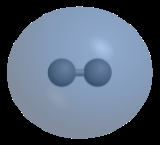Molecular orbitalEncyclopedia
In chemistry
Chemistry
Chemistry is the science of matter, especially its chemical reactions, but also its composition, structure and properties. Chemistry is concerned with atoms and their interactions with other atoms, and particularly with the properties of chemical bonds....

, a molecular orbital (or MO) is a mathematical function
Function (mathematics)
In mathematics, a function associates one quantity, the argument of the function, also known as the input, with another quantity, the value of the function, also known as the output. A function assigns exactly one output to each input. The argument and the value may be real numbers, but they can...

describing the wave-like behavior of an electron
Electron
The electron is a subatomic particle with a negative elementary electric charge. It has no known components or substructure; in other words, it is generally thought to be an elementary particle. An electron has a mass that is approximately 1/1836 that of the proton...

in a molecule
Molecule
A molecule is an electrically neutral group of at least two atoms held together by covalent chemical bonds. Molecules are distinguished from ions by their electrical charge...

. This function can be used to calculate chemical and physical properties such as the probability of finding an electron
Electron
The electron is a subatomic particle with a negative elementary electric charge. It has no known components or substructure; in other words, it is generally thought to be an elementary particle. An electron has a mass that is approximately 1/1836 that of the proton...

in any specific region. The term "orbital" was first used in English by Robert S. Mulliken
Robert S. Mulliken
Robert Sanderson Mulliken was an American physicist and chemist, primarily responsible for the early development of molecular orbital theory, i.e. the elaboration of the molecular orbital method of computing the structure of molecules. Dr. Mulliken received the Nobel Prize for chemistry in 1966...

as the English translation of Schrödinger
Erwin Schrödinger
Erwin Rudolf Josef Alexander Schrödinger was an Austrian physicist and theoretical biologist who was one of the fathers of quantum mechanics, and is famed for a number of important contributions to physics, especially the Schrödinger equation, for which he received the Nobel Prize in Physics in 1933...

's 'Eigenfunktion'. It has since been equated with the "region" generated with the function. Molecular orbitals are usually constructed by combining atomic orbital
Atomic orbital
An atomic orbital is a mathematical function that describes the wave-like behavior of either one electron or a pair of electrons in an atom. This function can be used to calculate the probability of finding any electron of an atom in any specific region around the atom's nucleus...

s or hybrid orbitals from each atom
Atom
The atom is a basic unit of matter that consists of a dense central nucleus surrounded by a cloud of negatively charged electrons. The atomic nucleus contains a mix of positively charged protons and electrically neutral neutrons...

of the molecule, or other molecular orbitals from groups of atoms. They can be quantitatively calculated using the Hartree-Fock or Self-Consistent Field (SCF) methods.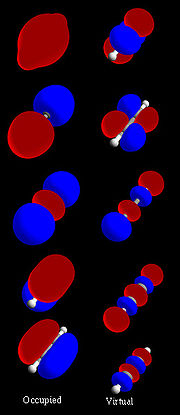## Overview

Molecular orbitals (MOs) represent regions in a molecule
Molecule
A molecule is an electrically neutral group of at least two atoms held together by covalent chemical bonds. Molecules are distinguished from ions by their electrical charge...

where an electron
Electron
The electron is a subatomic particle with a negative elementary electric charge. It has no known components or substructure; in other words, it is generally thought to be an elementary particle. An electron has a mass that is approximately 1/1836 that of the proton...

is likely to be found. Molecular orbitals are obtained from the combination of atomic orbitals, which predict the location of an electron in an atom. A molecular orbital can specify the electron configuration
Electron configuration
In atomic physics and quantum chemistry, electron configuration is the arrangement of electrons of an atom, a molecule, or other physical structure...

of a molecule: the spatial distribution and energy of one (or one pair of) electron(s). Most commonly an MO is represented as a linear combination of atomic orbitals
Linear combination of atomic orbitals molecular orbital method
A linear combination of atomic orbitals or LCAO is a quantum superposition of atomic orbitals and a technique for calculating molecular orbitals in quantum chemistry. In quantum mechanics, electron configurations of atoms are described as wavefunctions...

(the LCAO-MO method), especially in qualitative or very approximate usage. They are invaluable in providing a simple model of bonding in molecules, understood through molecular orbital theory
Molecular orbital theory
In chemistry, molecular orbital theory is a method for determining molecular structure in which electrons are not assigned to individual bonds between atoms, but are treated as moving under the influence of the nuclei in the whole molecule...

.
Most present-day methods in computational chemistry
Computational chemistry
Computational chemistry is a branch of chemistry that uses principles of computer science to assist in solving chemical problems. It uses the results of theoretical chemistry, incorporated into efficient computer programs, to calculate the structures and properties of molecules and solids...

begin by calculating the MOs of the system. A molecular orbital describes the behavior of one electron in the electric field generated by the nuclei and some average distribution of the other electrons. In the case of two electrons occupying the same orbital, the Pauli principle demands that they have opposite spin. Necessarily this is an approximation, and highly accurate descriptions of the molecular electronic wave function do not have orbitals (see configuration interaction
Configuration interaction
Configuration interaction is a post-Hartree–Fock linear variational method for solving the nonrelativistic Schrödinger equation within the Born–Oppenheimer approximation for a quantum chemical multi-electron system. Mathematically, configuration simply describes the linear combination...

).

## Formation of Molecular Orbitals

Molecular orbitals arise from allowed interactions between atomic orbital
Atomic orbital
An atomic orbital is a mathematical function that describes the wave-like behavior of either one electron or a pair of electrons in an atom. This function can be used to calculate the probability of finding any electron of an atom in any specific region around the atom's nucleus...

s, which are allowed if the symmetries (determined from group theory
Group theory
In mathematics and abstract algebra, group theory studies the algebraic structures known as groups.The concept of a group is central to abstract algebra: other well-known algebraic structures, such as rings, fields, and vector spaces can all be seen as groups endowed with additional operations and...

) of the atomic orbitals are compatible with each other. Efficiency of atomic orbital interactions is determined from the overlap (a measure of how well two orbitals constructively interact with one another) between two atomic orbitals, which is significant if the atomic orbitals are close in energy. Finally, the number of molecular orbitals that form must equal the number of atomic orbitals in the atoms being combined to form the molecule.

## Qualitative discussion

For an imprecise, but qualitatively useful, discussion of the molecular structure, the molecular orbitals can be obtained from the "Linear combination of atomic orbitals molecular orbital method
Linear combination of atomic orbitals molecular orbital method
A linear combination of atomic orbitals or LCAO is a quantum superposition of atomic orbitals and a technique for calculating molecular orbitals in quantum chemistry. In quantum mechanics, electron configurations of atoms are described as wavefunctions...

" ansatz
Ansatz
Ansatz is a German noun with several meanings in the English language.It is widely encountered in physics and mathematics literature.Since ansatz is a noun, in German texts the initial a of this word is always capitalised.-Definition:...

. Here, the molecular orbitals are expressed as linear combination
Linear combination
In mathematics, a linear combination is an expression constructed from a set of terms by multiplying each term by a constant and adding the results...

s of atomic orbital
Atomic orbital
An atomic orbital is a mathematical function that describes the wave-like behavior of either one electron or a pair of electrons in an atom. This function can be used to calculate the probability of finding any electron of an atom in any specific region around the atom's nucleus...

s.

### Linear combinations of atomic orbitals (LCAO)

Molecular orbitals were first introduced by Friedrich Hund
Friedrich Hund
Friedrich Hermann Hund was a German physicist from Karlsruhe known for his work on atoms and molecules.Hund worked at the Universities of Rostock, Leipzig, Jena, Frankfurt am Main, and Göttingen....

and Robert S. Mulliken
Robert S. Mulliken
Robert Sanderson Mulliken was an American physicist and chemist, primarily responsible for the early development of molecular orbital theory, i.e. the elaboration of the molecular orbital method of computing the structure of molecules. Dr. Mulliken received the Nobel Prize for chemistry in 1966...

in 1927 and 1928. The linear combination of atomic orbitals
Linear combination of atomic orbitals molecular orbital method
A linear combination of atomic orbitals or LCAO is a quantum superposition of atomic orbitals and a technique for calculating molecular orbitals in quantum chemistry. In quantum mechanics, electron configurations of atoms are described as wavefunctions...

or "LCAO" approximation for molecular orbitals was introduced in 1929 by Sir John Lennard-Jones
John Lennard-Jones
Sir John Edward Lennard-Jones KBE, FRS was a mathematician who was a professor of theoretical physics at Bristol University, and then of theoretical science at Cambridge University...

.. His ground-breaking paper showed how to derive the electronic structure of the fluorine
Fluorine
Fluorine is the chemical element with atomic number 9, represented by the symbol F. It is the lightest element of the halogen column of the periodic table and has a single stable isotope, fluorine-19. At standard pressure and temperature, fluorine is a pale yellow gas composed of diatomic...

and oxygen
Oxygen
Oxygen is the element with atomic number 8 and represented by the symbol O. Its name derives from the Greek roots ὀξύς and -γενής , because at the time of naming, it was mistakenly thought that all acids required oxygen in their composition...

molecules from quantum principles. This qualitative approach to molecular orbital theory is part of the start of modern quantum chemistry
Quantum chemistry
Quantum chemistry is a branch of chemistry whose primary focus is the application of quantum mechanics in physical models and experiments of chemical systems...

.
Linear combinations of atomic orbitals (LCAO) can be used to estimate the molecular orbitals that are formed upon bonding between the molecule’s constituent atoms. Similar to an atomic orbital, a Schrodinger equation, which describes the behavior of an electron, can be constructed for a molecular orbital as well. Linear combinations of atomic orbitals, or the sums and differences of the atomic wavefunctions, provide approximate solutions to the molecular Schrodinger equations. For simple diatomic molecules, the obtained wavefunctions are represented mathematically by the equations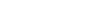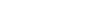where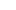and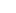are the molecular wavefunctions for the bonding and antibonding molecular orbitals, respectively,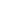and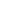are the atomic wavefunctions from atoms a and b, respectively, and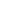and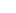are adjustable coefficients. These coefficients can be positive or negative, depending on the energies and symmetries of the individual atomic orbitals. As the two atoms become closer together, their atomic orbitals overlap to produce areas of high electron density, and, as a consequence, molecular orbitals are formed between the two atoms. The atoms are held together by the electrostatic attraction between the positively charged nuclei and the negatively charged electrons occupying bonding molecular orbitals.

### Bonding, Antibonding, and Nonbonding MOs

When atomic orbitals interact, the resulting molecular orbital can be of three types: bonding, antibonding, or nonbonding.

Bonding MOs:
• Bonding interactions between atomic orbitals are constructive (in-phase) interactions.
• Bonding MOs are lower in energy than the atomic orbitals that combine to produce them.

Antibonding MOs:
• Antibonding interactions between atomic orbitals are destructive (out-of-phase) interactions.
• Antibonding MOs are higher in energy than the atomic orbitals that combine to produce them.

Nonbonding MOs:
• Nonbonding MOs are the result of no interaction between atomic orbitals because of lack of compatible symmetries.
• Nonbonding MOs will have the same energy as the atomic orbitals of one of the atoms in the molecule.

### Sigma and Pi Labels for MOs

The type of interaction between atomic orbitals can be further categorized by the symmetry labels: σ (sigma) and π (pi).

#### σ-symmetry

A MO with σ-symmetry results from the interaction of either two atomic s-orbitals or two atomic pz-orbitals. A MO will have σ-symmetry if the orbital is symmetrical with respect to the axis joining the two nuclear centers, the internuclear axis. This means that rotation of the MO about the internuclear axis does not result in a phase change. A σ*-orbital, sigma antibonding orbital, also maintains the same phase when rotated about the internuclear axis. The σ*-orbital has a nodal plane that is between the nuclei and perpendicular to the internuclear axis.

#### π -symmetry

A MO with π –symmetry results from the interaction of either two atomic px-orbitals or py-orbitals. A MO will have π-symmetry if the orbital is asymmetrical with respect to rotation about the internuclear axis. This means that rotation of the MO about the internuclear axis will result in a phase change. A π*-orbital, pi antibonding orbital, will also produce a phase change when rotated about the internuclear axis. The π *-orbital also has a nodal plane between the nuclei.

For molecules that possess a center of inversion (centrosymmetric molecules
Centrosymmetry
The term centrosymmetric, as generally used in crystallography, refers to a space group which contains an inversion center as one of its symmetry elements. In such a space group, for every point in the unit cell there is an indistinguishable point...

) there are additional labels of symmetry that can be applied to molecular orbitals.
Centrosymmetric molecules include:
• Homonuclear
Homonuclear molecule
Homonuclear molecules, or homonuclear species, are molecules composed of only one type of element. Homonuclear molecules may consist of various numbers of atoms, depending on the element's properties. Some elements form molecules of more than one size. Noble gases rarely form bonds, so they only...

, X2
• Octahedral
Octahedral molecular geometry
In chemistry, octahedral molecular geometry describes the shape of compounds where in six atoms or groups of atoms or ligands are symmetrically arranged around a central atom, defining the vertices of an octahedron...

, EX6
• Square planar, EX4.

Non-centrosymmetric molecules include:
• Heteronuclear
Heteronuclear molecule
Heteronuclear molecules, or heteronuclear species, are molecules composed of more than one type of element....

, XY
• Tetrahedral
Tetrahedral molecular geometry
In a tetrahedral molecular geometry a central atom is located at the center with four substituents that are located at the corners of a tetrahedron. The bond angles are cos−1 ≈ 109.5° when all four substituents are the same, as in CH4. This molecular geometry is common throughout the first...

, EX4.

If inversion through the center of symmetry in a molecule results in the same phases for the molecular orbital, then the MO is said to have gerade, g, symmetry.
If inversion through the center of symmetry in a molecule results in a phase change for the molecular orbital, then the MO is said to have ungerade, u, symmetry.
For a bonding MO with σ-symmetry, the orbital can be labeled σg, and for an antibonding MO with σ-symmetry the orbital can be labeled σu, because inversion through the center of symmetry for Figure 4(b) would produce a sign change.
For a bonding MO with π -symmetry the orbital can be labeled πu because inversion through the center of symmetry for Figure 5(c) would produce a sign change. However, for an antibonding MO with π -symmetry the orbital can be labeled πg because inversion through the center of symmetry for Figure 5(d) would not produce a sign change.

### MO diagrams

The qualitative approach of MO uses a molecular orbital diagram to visualize bonding interactions in a molecule. In this type of diagram, the molecular orbitals are represented by horizontal lines; the higher a line the higher the energy of the orbital, and degenerate orbitals are placed on the same level with a space between them. Then, the electrons to be placed in the molecular orbitals are slotted in one by one, keeping in mind the Pauli exclusion principle and Hund's rule of maximum multiplicity (only 2 electrons, having opposite spins, per orbital; place as many unpaired electrons on one energy level as possible before starting to pair them). For more complicated molecules, the wave mechanics approach loses utility in a qualitative understanding of bonding (although is still necessary for a quantitative approach).
Some properties:
• A basis set of orbitals includes those atomic orbitals that are available for molecular orbital interactions, which may be bonding or antibonding
• The number of molecular orbitals is equal to the number of atomic orbitals included in the linear expansion or the basis set
• If the molecule has some symmetry, the degenerate atomic orbitals (with the same atomic energy) are grouped in linear combinations (called symmetry-adapted atomic orbitals (SO)), which belong to the representation
Representation theory of finite groups
In mathematics, representation theory is a technique for analyzing abstract groups in terms of groups of linear transformations. See the article on group representations for an introduction...

of the symmetry group
Symmetry group
The symmetry group of an object is the group of all isometries under which it is invariant with composition as the operation...

, so the wave functions that describe the group are known as symmetry-adapted linear combinations (SALC).
• The number of molecular orbitals belonging to one group representation is equal to the number of symmetry-adapted atomic orbitals belonging to this representation
• Within a particular representation
Representation theory of finite groups
In mathematics, representation theory is a technique for analyzing abstract groups in terms of groups of linear transformations. See the article on group representations for an introduction...

, the symmetry-adapted atomic orbitals mix more if their atomic energy level
Energy level
A quantum mechanical system or particle that is bound -- that is, confined spatially—can only take on certain discrete values of energy. This contrasts with classical particles, which can have any energy. These discrete values are called energy levels...

s are closer.

#### Orbital degeneracy

Molecular orbitals are said to be degenerate if they have the same energy. For example, in the homonuclear diatomic molecules of the first ten elements, the molecular orbitals derived from the px and the py atomic orbitals result in two degenerate bonding orbitals (of low energy) and two degenerate antibonding orbitals (of high energy).

#### Ionic bonds

When the energy difference between the atomic orbitals of two atoms is quite large, one atom's orbitals contribute almost entirely to the bonding orbitals, and the others atom’s orbitals contribute almost entirely to the antibonding orbitals. Thus, the situation is effectively that some electrons have been transferred from one atom to the other. This is called an (mostly) ionic bond
Ionic bond
An ionic bond is a type of chemical bond formed through an electrostatic attraction between two oppositely charged ions. Ionic bonds are formed between a cation, which is usually a metal, and an anion, which is usually a nonmetal. Pure ionic bonding cannot exist: all ionic compounds have some...

.

#### Bond order and length

The bond order, or number of bonds, of a molecule can be determined by combining the number of electrons in bonding and antibonding molecular orbitals as follows:

Bond order = 0.5*[(number of electrons in bonding orbitals) - (number of electrons in antibonding orbitals)]

For example, N2, with eight electrons in bonding orbitals and two electrons in antibonding orbitals, has a bond order of three, which constitutes a triple bond.
Bond length is inversely proportional to bond order. Data illustrating this principle for diatomic species of elements in the second row of the periodic table are shown in Figure 6.

Note that although Be2 has a bond order of 0 according to MO, there is experimental evidence of a highly unstable Be2 compound having a bond length of 245 pm and bond energy of 10kJ/mol. .

#### HOMO and LUMO

The highest occupied molecular orbital and lowest unoccupied molecular orbital are often referred to as the HOMO and LUMO, respectively. The difference of the energies of the HOMO and LUMO, termed the band gap
Band gap
In solid state physics, a band gap, also called an energy gap or bandgap, is an energy range in a solid where no electron states can exist. In graphs of the electronic band structure of solids, the band gap generally refers to the energy difference between the top of the valence band and the...

, can sometimes serve as a measure of the excitability
Excited state
Excitation is an elevation in energy level above an arbitrary baseline energy state. In physics there is a specific technical definition for energy level which is often associated with an atom being excited to an excited state....

of the molecule: The smaller the energy the more easily it will be excited. (more information forthcoming)

### Homonuclear diatomics

Homonuclear diatomic MOs contain equal contributions from each atomic orbital in the basis set. This is shown in the homonuclear diatomic MO diagrams for H2, He2, and Li2, all of which containing symmetric orbitals.

#### H2

As a simple MO example consider the hydrogen
Hydrogen
Hydrogen is the chemical element with atomic number 1. It is represented by the symbol H. With an average atomic weight of , hydrogen is the lightest and most abundant chemical element, constituting roughly 75% of the Universe's chemical elemental mass. Stars in the main sequence are mainly...

molecule, H2 (see molecular orbital diagram), with the two atoms labelled H' and H". The lowest-energy atomic orbitals, 1s' and 1s", do not transform according to the symmetries of the molecule. However, the following symmetry adapted atomic orbitals do:
1s' - 1s" Antisymmetric combination: negated by reflection, unchanged by other operations Symmetric combination: unchanged by all symmetry operations

The symmetric combination (called a bonding orbital) is lower in energy than the basis orbitals, and the antisymmetric combination (called an antibonding
Antibonding
Antibonding is a type of chemical bonding. An antibonding orbital is a form of molecular orbital that is located outside the region of two distinct nuclei...

orbital) is higher. Because the H2 molecule has two electrons, they can both go in the bonding orbital, making the system lower in energy (and, hence, more stable) than two free hydrogen atoms. This is called a covalent bond
Covalent bond
A covalent bond is a form of chemical bonding that is characterized by the sharing of pairs of electrons between atoms. The stable balance of attractive and repulsive forces between atoms when they share electrons is known as covalent bonding....

. The bond order is equal to the number of bonding electrons minus the number of antibonding electrons, divided by 2. In this example, there are 2 electrons in the bonding orbital and none in the antibonding orbital; the bond order is 1, and there is a single bond between the two hydrogen atoms.

#### He2

On the other hand, consider the hypothetical molecule of He2 (see molecular orbital diagram) with the atoms labeled He' and He". Again, the lowest-energy atomic orbitals, 1s' and 1s", do not transform according to the symmetries of the molecule, while the following symmetry adapted atomic orbitals do:
1s' - 1s" Antisymmetric combination: negated by reflection, unchanged by other operations Symmetric combination: unchanged by all symmetry operations

Similar to the molecule H2, the symmetric combination (called a bonding orbital) is lower in energy than the basis orbitals, and the antisymmetric combination (called an antibonding orbital) is higher. However, in its neutral ground state, each helium atom contains two electrons in its 1s orbital, combining for a total of four electrons. Two electrons fill the lower-energy bonding orbital, while the remaining two fill the higher-energy antibonding orbital. Thus, the resulting electron density around the molecule does not support the formation of a bond between the two atoms (called a sigma bond); therefore, the molecule does not exist. Another way of looking at it is that there are two bonding electrons and two antibonding electrons; therefore, the bond order is 0 and no bond exists. This is shown in Figure 10(a) in which two electrons occupy the σg(1s) bonding orbital and two electrons occupy the σu*(1s) antibonding orbital.

#### Li2

Dilithium
Dilithium
Dilithium, Li2, is a diatomic molecule comprising two lithium atoms covalently bonded together. Li2 is known in the gas phase. It has a bond order of 1, an internuclear separation of 267.3 pm and a bond energy of 101 kJ mol−1....

Li2 is formed from the overlap of the 1s and 2s atomic orbitals (the basis set) of two Li atoms. Each Li atom contributes three electrons for bonding interactions, and the six electrons fill the three MOs of lowest energy, σg(1s), σu*(1s), and σg(2s) in the MO diagram in Figure 10(b). Using the equation for bond order
Bond order
Bond order is the number of chemical bonds between a pair of atoms. For example, in diatomic nitrogen N≡N the bond order is 3, while in acetylene H−C≡C−H the bond order between the two carbon atoms is also 3, and the C−H bond order is 1. Bond order gives an indication to the stability of a bond....

, it is found that dilithium has a bond order of one, a single bond.

#### Noble gases

Considering a hypothetical molecule of He2, since the basis set of atomic orbitals is the same as in the case of H2, we find that both the bonding and antibonding orbitals are filled, so there is no energy advantage to the pair. HeH would have a slight energy advantage, but not as much as H2 + 2 He, so the molecule exists only a short while. In general, we find that atoms such as He that have completely full energy shells rarely bond with other atoms. Except for short-lived Van der Waals complexes, there are very few noble gas compound
Noble gas compound
Noble gas compounds are chemical compounds that include an element from Group 18 of the periodic table, the noble gases.-History and background:...

s known.

### Heteronuclear diatomics

While MOs for homonuclear diatomic molecules contain equal contributions from each interacting atomic orbital, MOs for heteronuclear diatomics contain different atomic orbital contributions. Orbital interactions to produce bonding or antibonding orbitals in heteronuclear diatomics occur if there is sufficient overlap between atomic orbitals as determined by their symmetries and similarity in orbital energies.

#### HF

In hydrogen fluoride
Hydrogen fluoride
Hydrogen fluoride is a chemical compound with the formula HF. This colorless gas is the principal industrial source of fluorine, often in the aqueous form as hydrofluoric acid, and thus is the precursor to many important compounds including pharmaceuticals and polymers . HF is widely used in the...

HF overlap between the H 1s and F 2s orbitals is allowed by symmetry but the difference in energy between the two atomic orbitals prevents them from interacting to create a molecular orbital. Overlap between the H 1s and F 2pz orbitals is also symmetry allowed, and these two atomic orbitals have a small energy separation. Thus, they interact, leading to creation of σ and σ* MOs and a molecule with a bond order of 1. Since HF is a non-centrosymmetric molecule, the symmetry labels g and u do not apply to its molecular orbitals.

## Quantitative approach

To obtain quantitative values for the molecular energy level
Energy level
A quantum mechanical system or particle that is bound -- that is, confined spatially—can only take on certain discrete values of energy. This contrasts with classical particles, which can have any energy. These discrete values are called energy levels...

s, one needs to have molecular orbitals that are such that the configuration interaction
Configuration interaction
Configuration interaction is a post-Hartree–Fock linear variational method for solving the nonrelativistic Schrödinger equation within the Born–Oppenheimer approximation for a quantum chemical multi-electron system. Mathematically, configuration simply describes the linear combination...

(CI) expansion converges fast towards the full CI
Full configuration interaction
Full configuration interaction is a linear variational approach which provides numerically exact solutions to the electronic time-independent, non-relativistic Schrödinger equation....

limit. The most common method to obtain such functions is the Hartree–Fock method, which expresses the molecular orbitals as eigenfunction
Eigenfunction
In mathematics, an eigenfunction of a linear operator, A, defined on some function space is any non-zero function f in that space that returns from the operator exactly as is, except for a multiplicative scaling factor. More precisely, one has...

s of the Fock operator. One usually solves this problem by expanding the molecular orbitals as linear combinations of Gaussian functions centered on the atomic nuclei
Atomic nucleus
The nucleus is the very dense region consisting of protons and neutrons at the center of an atom. It was discovered in 1911, as a result of Ernest Rutherford's interpretation of the famous 1909 Rutherford experiment performed by Hans Geiger and Ernest Marsden, under the direction of Rutherford. The...

(see linear combination of atomic orbitals
Linear combination of atomic orbitals molecular orbital method
A linear combination of atomic orbitals or LCAO is a quantum superposition of atomic orbitals and a technique for calculating molecular orbitals in quantum chemistry. In quantum mechanics, electron configurations of atoms are described as wavefunctions...

and basis set (chemistry)
Basis set (chemistry)
A basis set in chemistry is a set of functions used to create the molecular orbitals, which are expanded as a linear combination of such functions with the weights or coefficients to be determined. Usually these functions are atomic orbitals, in that they are centered on atoms. Otherwise, the...

). The equation for the coefficients of these linear combination
Linear combination
In mathematics, a linear combination is an expression constructed from a set of terms by multiplying each term by a constant and adding the results...

s is a generalized eigenvalue equation known as the Roothaan equations
Roothaan equations
The Roothaan equations are a representation of the Hartree-Fock equation in a non orthonormal basis set which can be of Gaussian-type or Slater-type. It applies to closed-shell molecules or atoms where all molecular orbitals or atomic orbitals, respectively, are doubly occupied. This is generally...

, which are in fact a particular representation of the Hartree-Fock equation. There are a number of programs in which quantum chemical calculations of MOs can be performed, including Spartan
Spartan (software)
SPARTAN is a molecular modeling and computational chemistry application from . It contains code for molecular mechanics, semi-empirical methods, ab initio models, density functional models, post-Hartree-Fock models, and thermochemical recipes including T1....

and HyperChem.

Simple accounts often suggest that experimental molecular orbital energies can be obtained by the methods of ultra-violet photoelectron spectroscopy for valence orbitals and X-ray photoelectron spectroscopy
X-ray photoelectron spectroscopy
X-ray photoelectron spectroscopy is a quantitative spectroscopic technique that measures the elemental composition, empirical formula, chemical state and electronic state of the elements that exist within a material...

for core orbitals. This, however, is incorrect as these experiments measure the ionization energy, the difference in energy between the molecule and one of the ions resulting from the removal of one electron. Ionization energies are linked approximately to orbital energies by Koopmans' theorem
Koopmans' theorem
Koopmans' theorem states that in closed-shell Hartree-Fock theory, the first ionization energy of a molecular system is equal to the negative of the orbital energy of the highest occupied molecular orbital...

. While the agreement between these two values can be close for some molecules, it can be very poor in other cases.